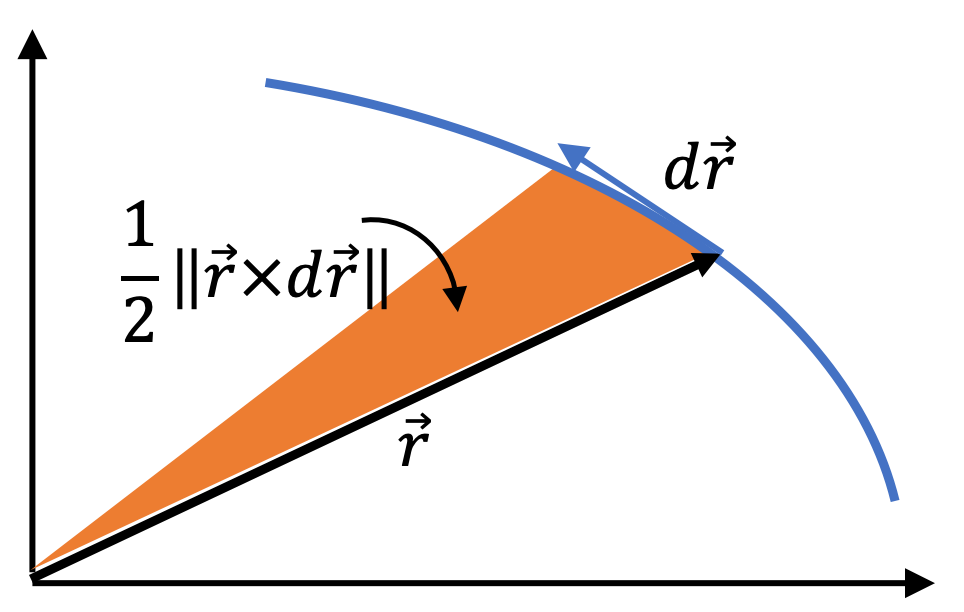## Section12.5Deriving Kepler's Second Law

###### (Calculus) Conservation of Angular Momentum in Sun-Planet System.

Kepler's second law is a consequence of the conservation of angular momentum. We look at the motion of one planet and the Sun, a two-body problem interacting with gravitational form. We will work in the CM frame, where the problem simplifies to the motion of one particle of mass equal to reduced mass.

\begin{equation} \mu\dfrac{d^2\vec r}{dt^2} = -G_N \dfrac{\mu\, M}{r^2}\, \hat u,\tag{12.5.1} \end{equation}

where $\hat u$ is unit vector pointed towards the origin, the radially inward direction. We can rewrite this as

\begin{equation} \dfrac{d(\mu \vec v)}{dt} = -G_N \dfrac{\mu\, M}{r^2}\, \hat u,\tag{12.5.2} \end{equation}

where $\vec v = d\vec r/ dt \text{.}$ The left side is the rate of change of momentum. Taking cross product with $\vec r$ on both sides we get

\begin{equation} \vec r \times \dfrac{d(\mu \vec v)}{dt} = -G_N \dfrac{\mu\, M}{r^2}\, \vec r \times \hat u.\label{eq-rcross-dmuvdt}\tag{12.5.3} \end{equation}

The right side is zero since there is $180^\circ$ angle between $\vec r$ and $\hat u\text{.}$ By moving $\vec r \times$ inside the $d/dt$ on the left side, we can show that

\begin{align*} \dfrac{d( \vec r \times \mu \vec v)}{dt} \amp =\dfrac{d\vec r}{dt} \times \mu \vec v + \vec r \times \dfrac{d(\mu \vec v)}{dt},\\ \amp = \vec v \times \mu \vec v + \vec r \times \dfrac{d(\mu \vec v)}{dt}\\ \amp = 0 + \vec r \times \dfrac{d(\mu \vec v)}{dt} \end{align*}

Therefore, Eq. (12.5.3) gives the following equation.

\begin{equation} \dfrac{d (\vec r \times \mu \vec v)}{dt} = 0.\tag{12.5.4} \end{equation}

Since $\vec r \times \mu \vec v$ is angular momentum of the relative motion, this is the statement of conservation of angular momentum. We can pull $\mu$ out, giving us the following equation obeyed by the trajectory $\vec r(t) \text{.}$

\begin{equation} \vec r \times \vec v = \vec c,\tag{12.5.5} \end{equation}

where $\vec c$ is a constant vector. Rewrite this as

\begin{equation} \vec r \times d\vec r = \vec c\, dt,\label{eq-kepler-cons-ang-mom-eqn-to-integrate}\tag{12.5.6} \end{equation}

and note that the magnitude of $\vec r \times d\vec r$ is the area of the rectangle formed by vectors $\vec r$ and $d \vec r\text{,}$ which is twice the area swept by vector $\vec r \text{.}$

\begin{equation} \vec r \times d\vec r = 2\times \text{Area swept by }\vec r.\tag{12.5.7} \end{equation}Figure 12.5.1. The position vector $\vec r$ sweeping out half the area given by the magnitude of $\vec r \times d\vec r\text{.}$ By conservation of angular momentum, it is shown that the magnitude of the integral $\int \vec r \times d\vec r$ is proportional to time. Therefore, we get the law that radial vector from the Sun to a planet sweeps out equal area in equal time. When we integrate Eq. (12.5.6) for any interval $t_i$ to $t_f\text{,}$ we find that

\begin{equation*} \int_{\vec r(t_i)}^{\vec r(f)} \vec r \times d\vec r = \vec c (t_f - t_i), \end{equation*}

where the left side is twice the area swept by vector $\vec r$ as shown in Figure 12.5.1. Writing the magnitudes only with $\Delta A$ for area swept and $\Delta t$ for time interval, we will get

\begin{equation*} \dfrac{\Delta A}{\Delta t} = c/2,\text{ a constant.} \end{equation*}

That is, the radial position vector of $\mu$ sweeps out equal area in equal time. Since mass of Sun is much greater than most planets, particle $\mu$ can be replaced by the planet alone, which is the observation made by Kepler in his second law of planetary motion. By Newton's analysis, we get more than Kepler's law - we see the precise variables we should look at when we are computing the area swept. Additionally, we see that Kepler's second law is a consequence of cnservation of angular momentum.